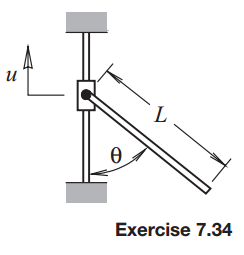### Create an Account

Already have account?

### Forgot Your Password ?

Home / Questions / The collar to which the bar is pinned is given a specified displacement ut The collar and ...

# The collar to which the bar is pinned is given a specified displacement ut The collar and the bar have equal mass m Use Lagranges equations to derive the equations of motion for this system

The collar to which the bar is pinned is given a specified displacement u(t). The collar and the bar have equal mass m. Use Lagrange’s equations to derive the equations of motion for this system.Jun 16 2020 View more View Less

#### Answer (Solved)Subscribe To Get Solution